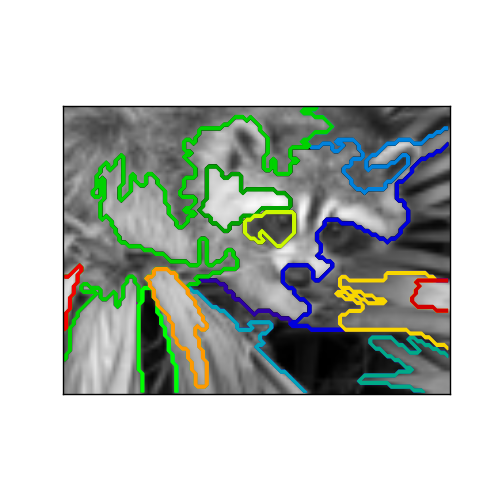# A demo of structured Ward hierarchical clustering on a raccoon face image¶

Compute the segmentation of a 2D image with Ward hierarchical clustering. The clustering is spatially constrained in order for each segmented region to be in one piece.

```# Author : Vincent Michel, 2010
#          Alexandre Gramfort, 2011

print(__doc__)

import time as time

import numpy as np
import scipy as sp

import matplotlib.pyplot as plt

from sklearn.feature_extraction.image import grid_to_graph
from sklearn.cluster import AgglomerativeClustering
from sklearn.utils.testing import SkipTest
from sklearn.utils.fixes import sp_version

if sp_version < (0, 12):
raise SkipTest("Skipping because SciPy version earlier than 0.12.0 and "
"thus does not include the scipy.misc.face() image.")
```

Generate data

```try:
face = sp.face(gray=True)
except AttributeError:
# Newer versions of scipy have face in misc
from scipy import misc
face = misc.face(gray=True)

# Resize it to 10% of the original size to speed up the processing
face = sp.misc.imresize(face, 0.10) / 255.

X = np.reshape(face, (-1, 1))
```

Define the structure A of the data. Pixels connected to their neighbors.

```connectivity = grid_to_graph(*face.shape)
```

Compute clustering

```print("Compute structured hierarchical clustering...")
st = time.time()
n_clusters = 15  # number of regions
connectivity=connectivity)
ward.fit(X)
label = np.reshape(ward.labels_, face.shape)
print("Elapsed time: ", time.time() - st)
print("Number of pixels: ", label.size)
print("Number of clusters: ", np.unique(label).size)
```

Out:

```  Compute structured hierarchical clustering...
Elapsed time:  0.635977983475
Number of pixels:  7752
Number of clusters:  15
```

Plot the results on an image

```plt.figure(figsize=(5, 5))
plt.imshow(face, cmap=plt.cm.gray)
for l in range(n_clusters):
plt.contour(label == l, contours=1,
colors=[plt.cm.spectral(l / float(n_clusters)), ])
plt.xticks(())
plt.yticks(())
plt.show()
```Total running time of the script: (0 minutes 1.023 seconds)

Download Python source code: `plot_face_ward_segmentation.py`